Next: Partial Waves Up: Scattering Theory Previous: Fundamental Equations

# Born Approximation

Equation (938) is not particularly useful, as it stands, because the quantity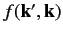depends on the unknown ket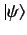. Recall thatis the solution of the integral equation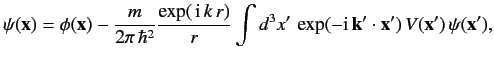(939)

where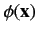is the wavefunction of the incident state. According to the above equation, the total wavefunction is a superposition of the incident wavefunction and lots of spherical waves emitted from the scattering region. The strength of the spherical wave emitted at a given point is proportional to the local value of the scattering potential,, as well as the local value of the wavefunction,.

Suppose that the scattering is not particularly strong. In this case, it is reasonable to suppose that the total wavefunction,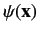, does not differ substantially from the incident wavefunction,. Thus, we can obtain an expression forby making the substitution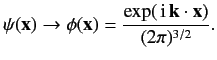(940)

This is called the Born approximation.

The Born approximation yields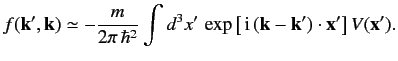(941)

Thus,is proportional to the Fourier transform of the scattering potentialwith respect to the wavevector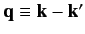.

For a spherically symmetric potential,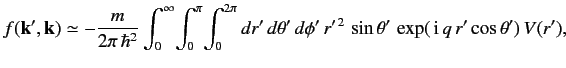(942)

giving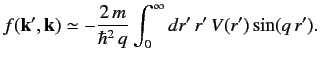(943)

Note thatis just a function offor a spherically symmetric potential. It is easily demonstrated that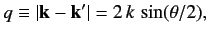(944)

where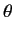is the angle subtended between the vectors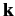and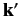. In other words,is the angle of scattering. Recall that the vectorsandhave the same length, as a consequence of energy conservation.

Consider scattering by a Yukawa potential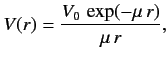(945)

where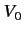is a constant, and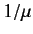measures the range'' of the potential. It follows from Equation (943) that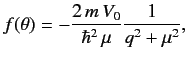(946)

because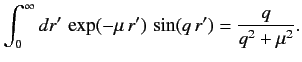(947)

Thus, in the Born approximation, the differential cross-section for scattering by a Yukawa potential is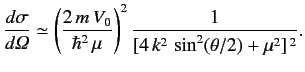(948)

The Yukawa potential reduces to the familiar Coulomb potential as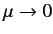, provided that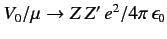. In this limit, the Born differential cross-section becomes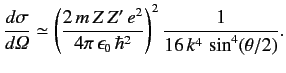(949)

Recall that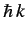is equivalent to, so the above equation can be rewritten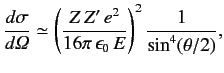(950)

where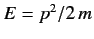is the kinetic energy of the incident particles. Equation (950) is identical to the classical Rutherford scattering cross-section formula.

The Born approximation is valid provided thatis not too different fromin the scattering region. It follows, from Equation (922), that the condition for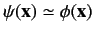in the vicinity of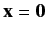is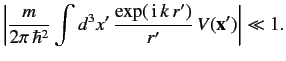(951)

Consider the special case of the Yukawa potential. At low energies, (i.e.,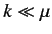) we can replace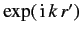by unity, giving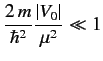(952)

as the condition for the validity of the Born approximation. The condition for the Yukawa potential to develop a bound state is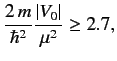(953)

whereis negative. Thus, if the potential is strong enough to form a bound state then the Born approximation is likely to break down. In the high-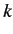limit, Equation (951) yields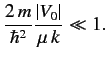(954)

This inequality becomes progressively easier to satisfy asincreases, implying that the Born approximation is more accurate at high incident particle energies.Next: Partial Waves Up: Scattering Theory Previous: Fundamental Equations
Richard Fitzpatrick 2013-04-08